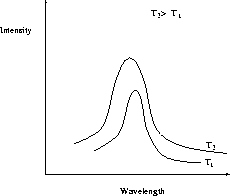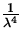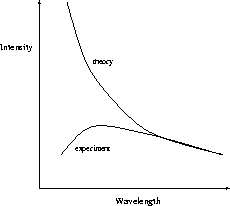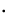Up until the late 1800's the wave picture of light was the prevalent theory, as it could explain most of the experiments done on light. However, there were a few notable exceptions. One such exception was that associated with blackbody radiation, which is the characteristic radiation that a body emits when heated. It was known that this radiation changes in nature as the temperature changes, and experiments on blackbodies'' (perfect absorbers and emitters) show the following typical curves of the intensity of the radiation (energy emitted per unit time per unit area) vs. the wavelength at a fixed temperature T , as in Fig. 27.1.When the wave picture of light was applied to this problem, however, it failed - it predicted that the intensity, I , for a given temperature should behave as

 I(1)

which agrees with the experimental data for long wavelengths but diverges for short wavelengths, unlike what really happens. This is illustrated in Fig. 27.2.In 1900 Planck devised a theory of blackbody radiation which gave good agreement for all wavelengths. In this theory the molecules of a body cannot have arbitrary energies but instead are quantized - the energies can only have discrete values. The magnitude of these energies is given by the formula

 E = nhf, (2)

where n = 0,1,2,... is an integer, f is the frequency of vibration of the molecule, and h is a constant, now called Planck's constant:

 h = 6.63 x 10- 34 Js . (3)

Furthermore, he postulated that when a molecule went from a higher energy state to a lower one it emitted a quanta (packet) of radiation, or photon, which carried away the excess energy.

With this photon picture, Planck was able to successfully explain the blackbody radiation curves, both at long and at short wavelengths. However, it was a radical departure from the conventional picture of light emitted from a blackbody, and at the time many people felt it was simply a calculational trick invented to obtain the right answer.Next: The Photoelectric Effect Up: Quantum Physics Previous: Quantum Physics